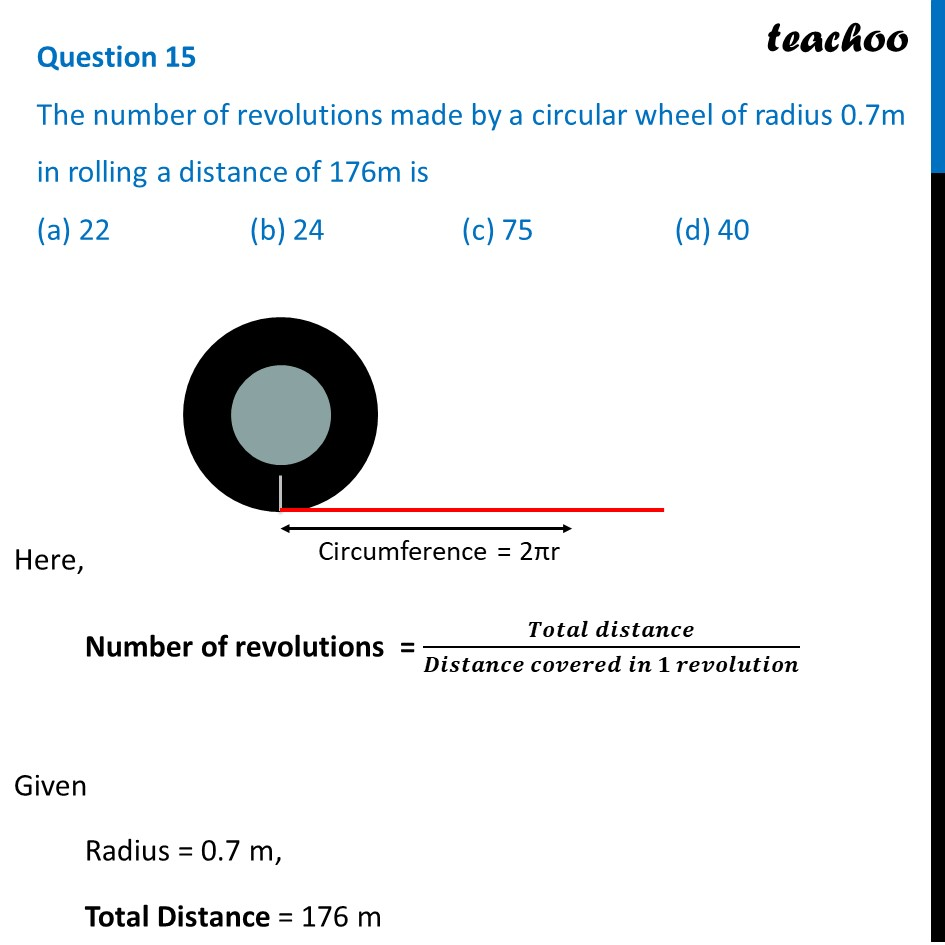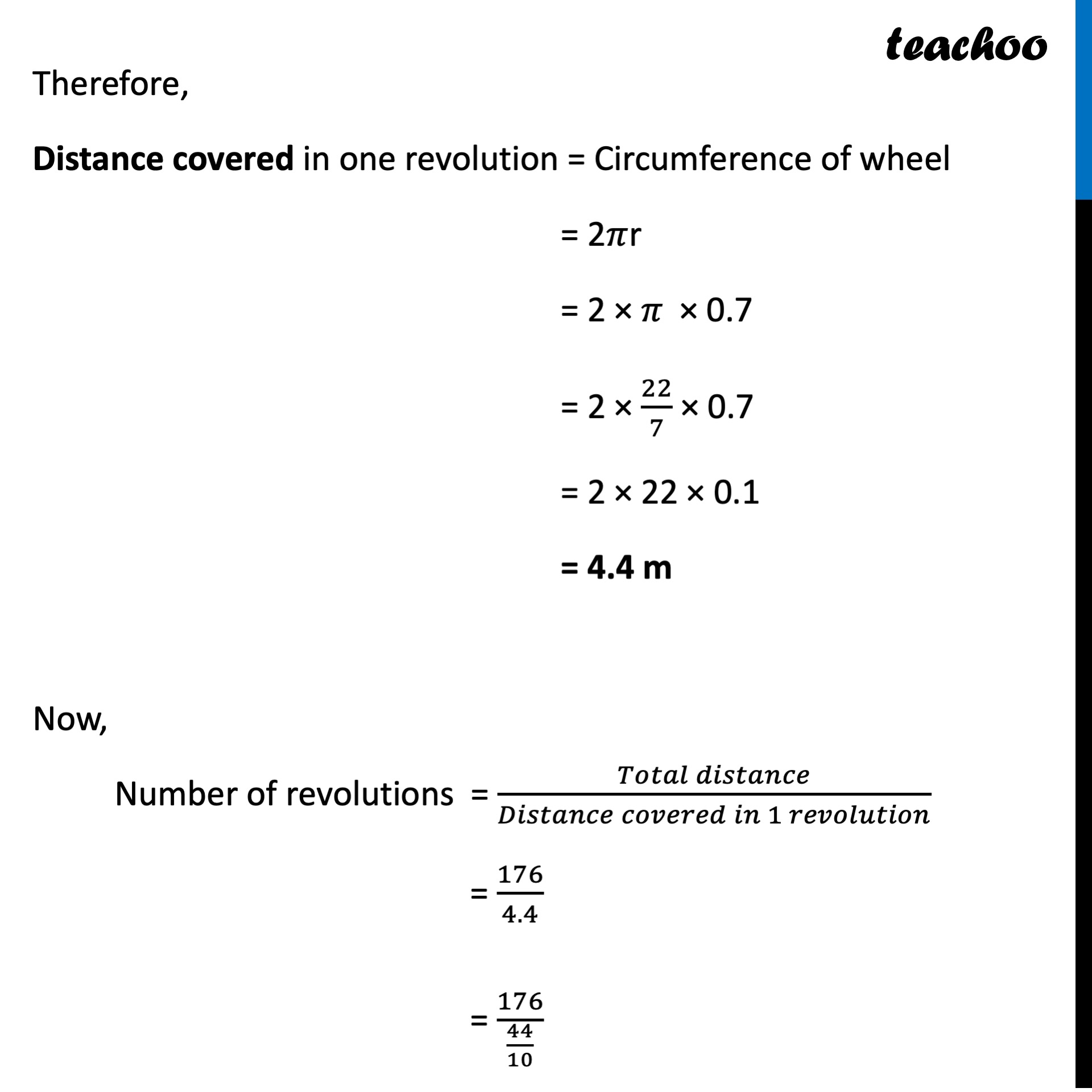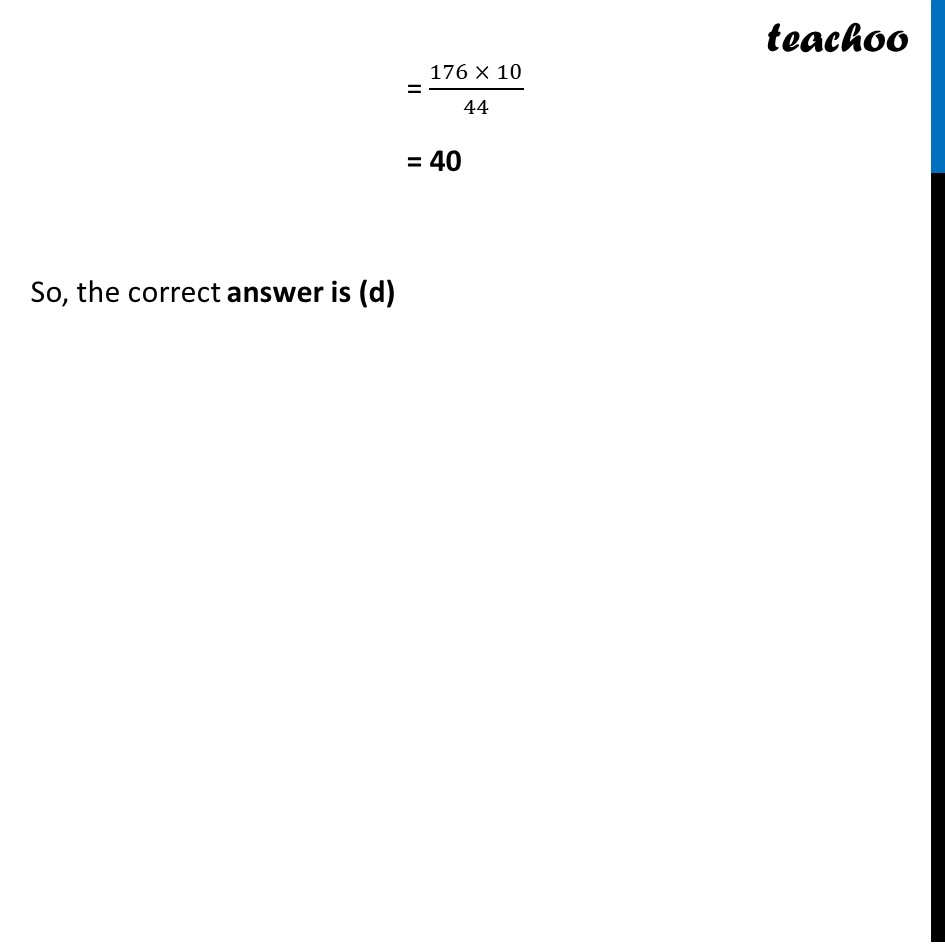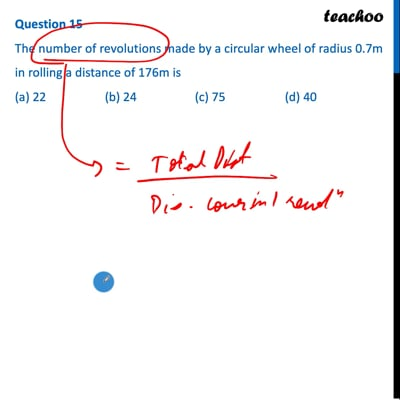CBSE Class 10 Sample Paper for 2022 Boards - Maths Standard [MCQ]

Class 10
Solutions of Sample Papers for Class 10 Boards

## (a) 22   (b) 24   (c) 75   (d) 40This video is only available for Teachoo black users

Get live Maths 1-on-1 Classs - Class 6 to 12

### Transcript

Question 15 The number of revolutions made by a circular wheel of radius 0.7m in rolling a distance of 176m is (a) 22 (b) 24 (c) 75 (d) 40 Here, Number of revolutions = (𝑻𝒐𝒕𝒂𝒍 𝒅𝒊𝒔𝒕𝒂𝒏𝒄𝒆)/(𝑫𝒊𝒔𝒕𝒂𝒏𝒄𝒆 𝒄𝒐𝒗𝒆𝒓𝒆𝒅 𝒊𝒏 𝟏 𝒓𝒆𝒗𝒐𝒍𝒖𝒕𝒊𝒐𝒏) Given Radius = 0.7 m, Total Distance = 176 m Therefore, Distance covered in one revolution = Circumference of wheel = 2𝜋r = 2 × 𝜋 × 0.7 = 2 × 22/7 × 0.7 = 2 × 22 × 0.1 = 4.4 m Now, Number of revolutions = (𝑇𝑜𝑡𝑎𝑙 𝑑𝑖𝑠𝑡𝑎𝑛𝑐𝑒)/(𝐷𝑖𝑠𝑡𝑎𝑛𝑐𝑒 𝑐𝑜𝑣𝑒𝑟𝑒𝑑 𝑖𝑛 1 𝑟𝑒𝑣𝑜𝑙𝑢𝑡𝑖𝑜𝑛) = 2 × 𝜋 × 0.7 = 176/(44/10) = (176 × 10)/44 = 40 So, the correct answer is (d)